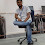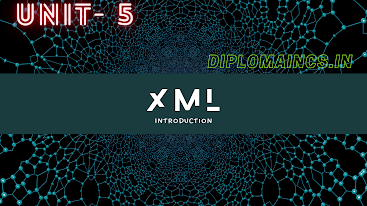## 8/24/20

### Introduction to programming through C

Computer Programming Through ‘C’

1.    INTRODUCTION TO PROGRAMMING (Part-1)
The Basic Model of Computation
Algorithms
Flowcharts
Programming Languages
Compilation
Testing and Debugging
Documentation:  Programming Style-Names, Documentation & Format, Refinement & Modularity

The basic model of computation: -
1. A computation model is a collection of values and operations.
2. A model of competition is the definition set of operation used in competition and their respective cost.
3. It is used for measuring the complicity of an algorithm in execution time and memory space.
4. Some basic steps of basic model of computation are as follows:
a. Formatting the problem and deciding the data types of to input.
b. Identifying the steps of computation that are necessary for getting the solution.
c. Identify decision points that is under what circumstances a particular operation is to  be  performed and when not to be performed
d. Knowing the expected results and very fine with the actual values.
5. Various types of basic model of computation they are as follows
a. The functional model
b. The logical model
c. The imperative model

Algorithm: -
1.   An algorithm is a procedure or set of rules or step by step instruction for solving a problem.
2.   The word algorithm means a process or set of rules to be followed in calculations or other problem-solving operations, especially by computer.
3.   Algorithm defines a set of instructions to be executed in a certain order to get the desired output.
4.   An algorithm can implement in more than one programming language because it generally created independent of major languages.
5.   Algorithm can easily perform calculation, data processing and accommodated reasoning task.
6.   Algorithms are just IT clean instruction that can be implemented in any language i.e. C, C++, Java etc. and the output will be the same as expected by the programmer.

Example of algorithm Exchange values of two variables

Step-1       START

Step-2       Declare variable a and b;

Step-5       temp=a;

Step-6       a=b;

Step-7       b=temp;

Step-8       Print the value of ‘a’ , ‘b’.

Step-9       END

Note: -  More algorithm

Algorithm shows that exchange of two values.
Start and Stop step is used for starting the algorithm and closing the algorithm respectfully.

Flowcharts: -
1.   A flowchart is a diagrammatical representation of an algorithm work process.
2.   A flowchart follows the work process of an algorithm, showing the setups, edges, boxes of their order which are connected through arrows.
3.   Flowchart uses simple geometric shapes like rectangle, diamond, oval, etc. to describe processes and geometric shapes are connects through arrow that shows relationships and process/dataflow.
4.   A programmer often use flowchart as a program planning tool to solve a problem.
5.   Flowcharting is a process of drawing a flowchart for an algorithm.
6.   There are some basic symbols or shapes used in
Flowchart designs:-

Programming Language: -
1. An artificial language that is design to express computations that can be performed by machine particularly a computer is known as a programming language.
2.  A programming language can be used to create programs that programs controls the behavior of a machine, to express algorithms as a mode of human communication.
3. As we know that a computer system is simply a machine therefore it cannot perform any work itself in order to make it functional different languages are developed that languages are known as programming languages or simply we can also say that a computer language.
4. If we want to write a program for a computer we must use a computer language.
5. Some major programming languages categories are
a. Machine language
b. Assembly language or symbolic
c. High level language

Machine languages:

1. Machine language is a sequence of instructions written in the form of binary numbers (0's and 1's) to which the computer responds directly.

2. Advantage of using machine language is that CPU directly understands machine instructions,   hence no translator is required.
3. Disadvantages of using machine languages are as follows

a.  Difficult to use

b.  Machine dependent

c.  Error-prone

d.  Difficult to develop and modify

Assembly language:

1. Assembly language contains instructions in English abbreviations called mnemonics which represents elementary operations of computer.

2. Assembly languages yes machine languages are referred to as low level languages.

3. In assembly language there is a assembler that is a translator that takes input in form of the Assembly language program and produces machine language code as its output

4. Advantage of using assembly language is to write a program in assembly language is more convenient than writing one in machine language.

5. Disadvantages of using assembly language are as follows

a. Machine dependent

b. Programming is difficult and time consuming

c. The program know all about the logical structure of the computer

High level language:

1. Language such as COBOL, FORTAN and BASIC are high level languages because all have instructions that are similar to human languages.

2. The programming style and the context it is easier to learn and implement in high level languages.

3. Compiler is a translator program which converts high level language instruction into machine language instructions.

4. Advantages of using high level languages are as follows:

b. Portability

c. Easy to debugging

d. Ease in the development of the software.

Part-2 of this unit i.e. Introduction To Programming (Part-2) will uploaded after some days. Keep update yourself.

1.Wow sir, you have given very good notes.
Thank you sir, you are helping us by giving notes.

1.Thank you much..!!

2.Thank you sir, it's too easy to understand.

1.You are welcome..!!

3.Thank you so much Sir, you are helping us by giving notes.

Please do not enter any spam link in the comment box and use English and Hindi language for comment.

## Latest Update

### Key Components of XML